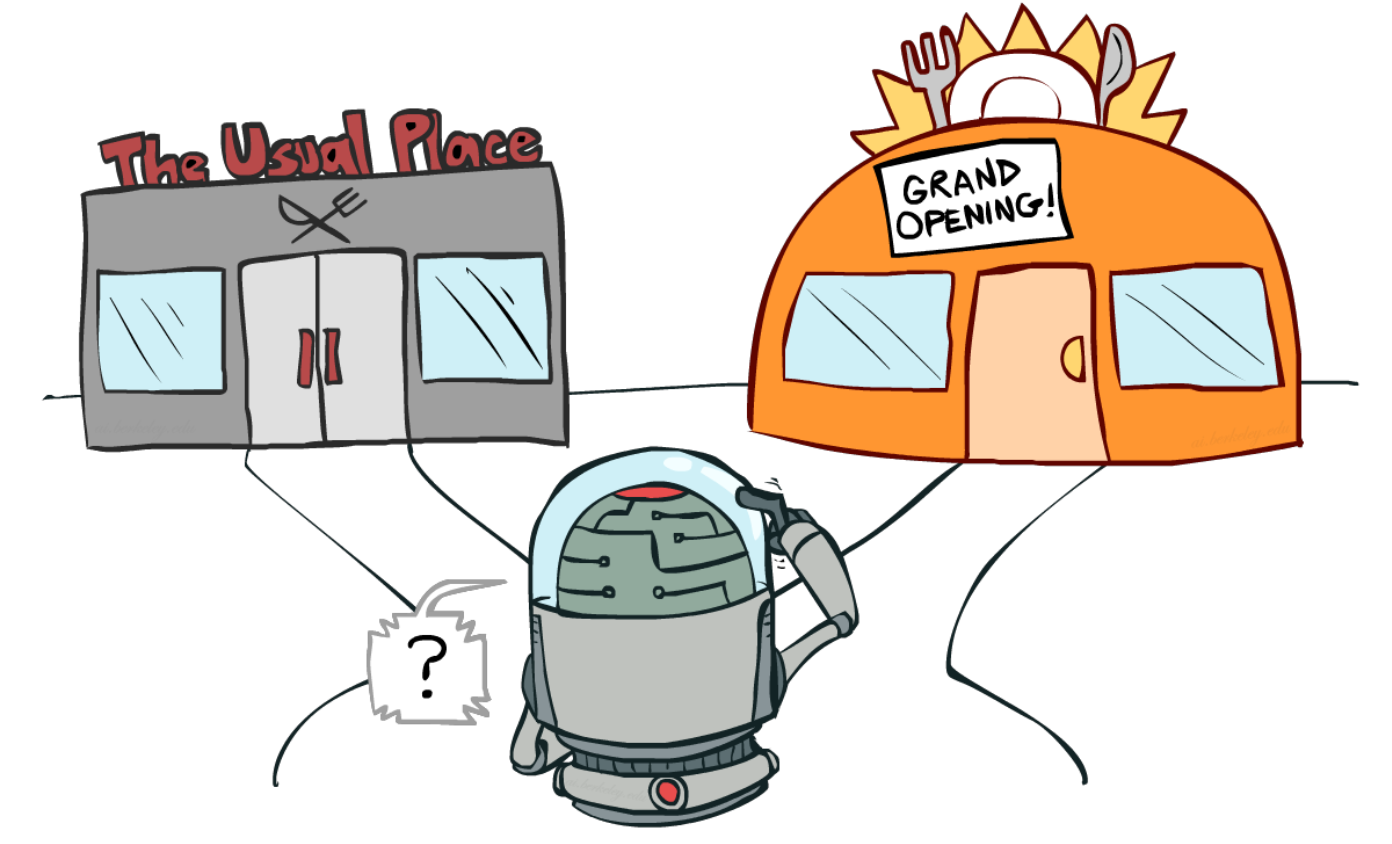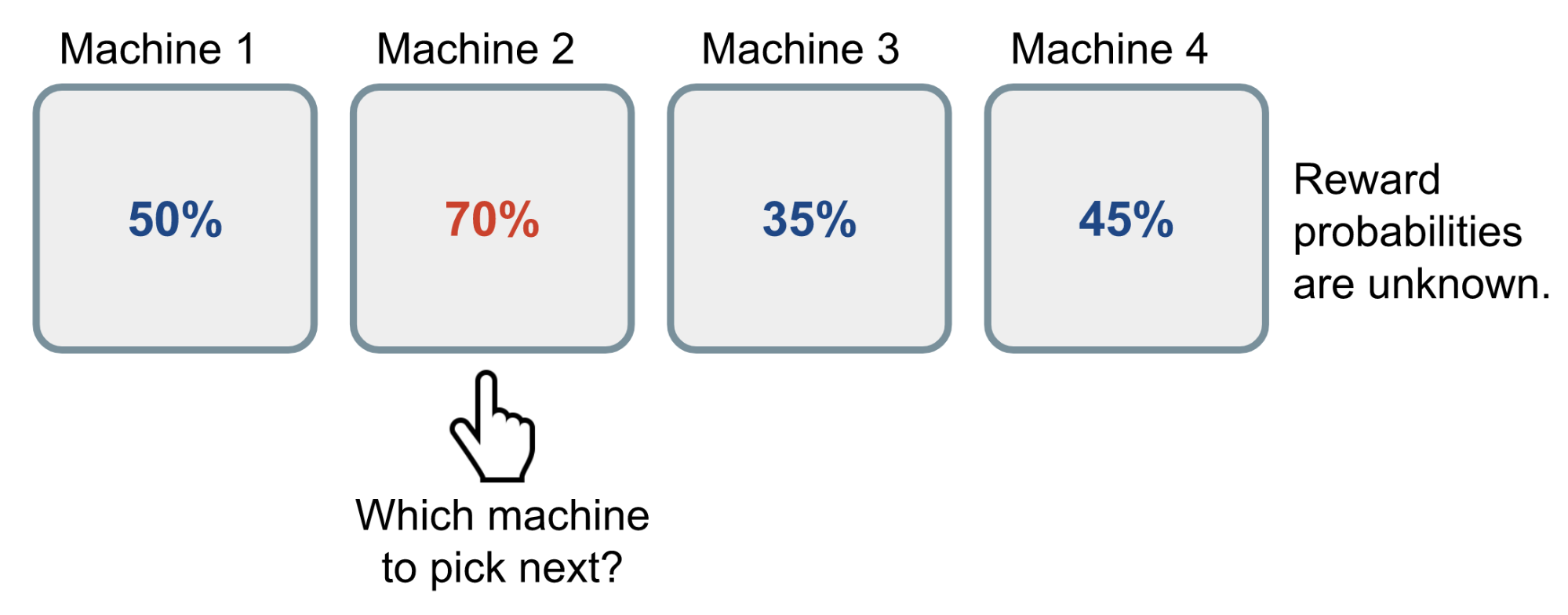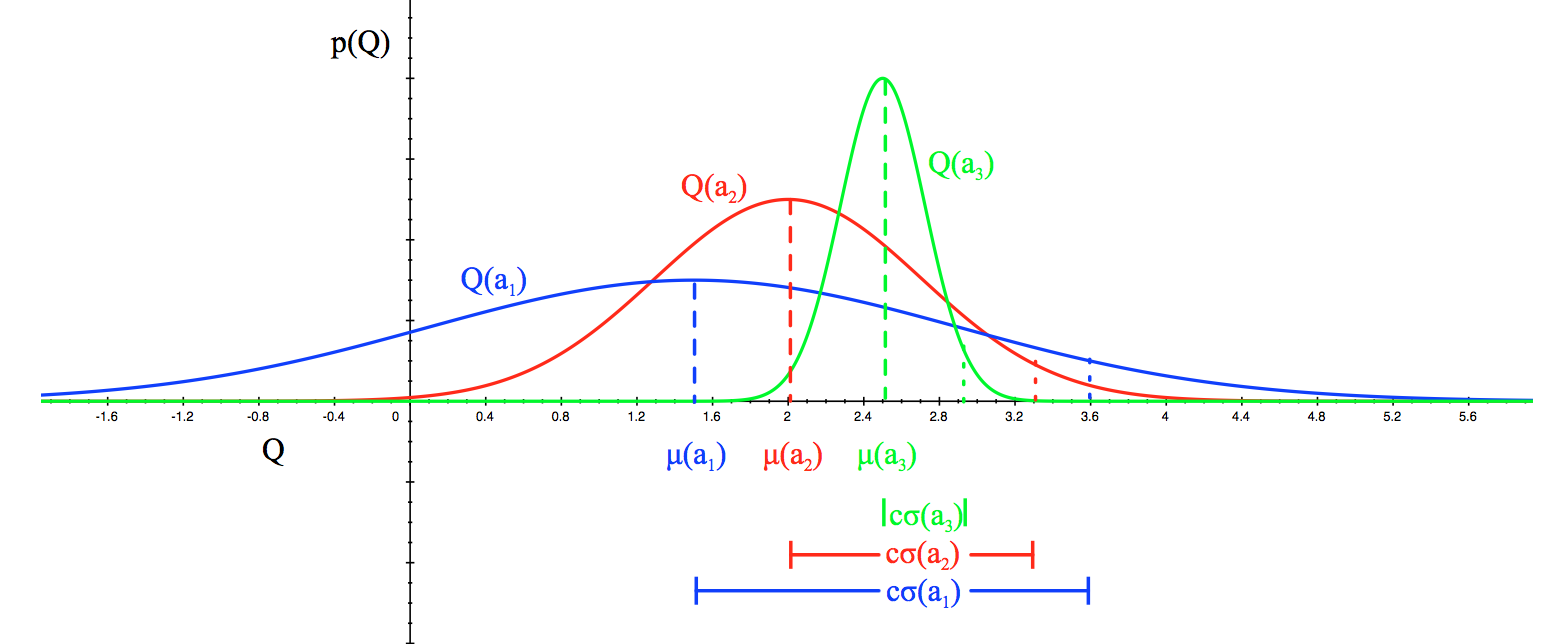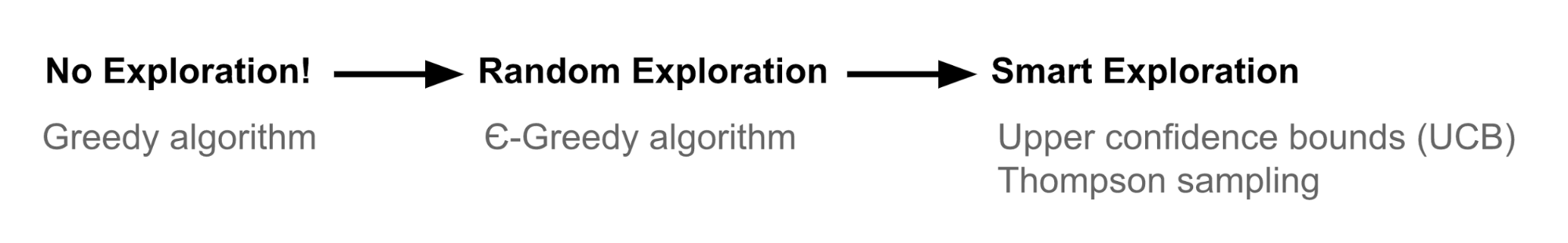# Exploration vs Exploitation

• 只去我常去的餐馆: 失去了探索其他更好的餐馆的机会
• 如果总是去新的餐馆吃饭, 那么你有可能吃到不合口味的饭菜# Multi-Armed Bandit

Multi-Armed Bandit, 多臂赌博机(K摇臂赌博机)问题关于探索-利用窘境的经典问题. 现在的问题是: 什么是最好的策略使得有最好的长期回报?(What is the best strategy to achieve highest long-term rewards?)Bernoulli multi-armed bandit 的工作原理: 在这个例子中, bandit 有 4 个 arm. , 没摇动一次就产生一个 reward, 为 0或1, 每个 arm 的回报为1的概率对于赌徒来说是未知的.

## 问题定义

Bernoulli multi-armed bandit 可以被描述为一个元组$\langle \mathcal{A}, \mathcal{R} \rangle$, 其中:

• 有$K$个机器, 有 reward 的概率为: $\left\{{\theta_1,\cdots,\theta_K}\right\}$
• 在任何时间步(time step)$t$, 都能够在一台机器上采取一个行为并且获得回报$r$
• $\mathcal{A}$是行为(action)的空间, 特定的行为$a$的值是期望的回报, $Q(a)=\mathbb{E}[r|a]=\theta$. 如果是在第i台机器上的行为, 则记为$Q(a_t)=\theta_i$
• $\mathcal{R}$是回报函数. 在 Bernoulli multi-armed bandit 中, 得到的回报$r$是一个随机(stochastic)的 fashion(?). 在时间步$t$, $r_t=\mathcal{R}(a_t)$在概率$Q(a_t)$下返回1, 否则返回0.

$$\theta^{*}=Q(a^{*})=\max_{a \in \mathcal{A}} Q(a) = \max_{1 \leq i \leq K} \theta_i$$

$$\mathcal{L}_T = \mathbb{E} \Big[ \sum_{t=1}^T \big( \theta^{*} - Q(a_t) \big) \Big]$$

## 赌博策略(Bandit Strategies)

• 不探索: 最原始(naive)的以及最坏的办法
• 随机探索(Exploration at random)
• 在不确定的情况下智能地选择偏好行为

# ε-greedy 算法

$$\hat{Q}_t(a) = \frac{1}{N_t(a)} \sum_{\tau=1}^t r_\tau \mathbb{I}[a_\tau = a]$$

# Upper Confidence Bounds

ε-greedy 算法是我们能够采取不清楚的行为, 但是引入的随机性(randomness)会造成我们最终会探索一些在过去已经证实为不好的行为. 为了阻止低效的探索, 我们需要及时地减小 ε 并且对高不确定性表示乐观也因此更加的偏向于选择还没有进行确定性(confident)的值估计. 也就是说我们偏向于用强潜力最优值来探索(we favor exploration of actions with a strong potential to have a optimal value).

Upper Confidence Bounds (UCB) 算法通过一个回报值的 upper confidence bound 来度量这个潜力, 记为$\hat{U}_t(a)$, 真正(只要是不足的估计就会有偏差)的值很有可能(with high probability)会不大于范围: $Q(a) \leq \hat{Q}_t(a) + \hat{U}_t(a)$. 上界(upper bound)$\hat{U}_t(a)$是$N_t(a)$的函数. 一个更大的尝试次数$N_t(a)$应该产生一个更小的界$\hat{U}(a)$.

$$a^{UCB}_t = \arg\max_{a \in \mathcal{A}} \hat{Q}_t(a) + \hat{U}_t(a)$$

## Hoeffding's Inequality

$$\mathbb{P} [ \mathbb{E}[X] > \overline{X}_t + u] \leq e^{-2tu^2}$$

• $r_t(a)$是随机变量
• $Q(a)$是期望(true mean)
• $\hat{Q}_t(a)$是采样均值
• $u$是 upper confidence bound, $u=U_t(a)$

$$\mathbb{P} [ Q(a) > \hat{Q}_t(a) + U_t(a)] \leq e^{-2t{U_t(a)}^2}$$

$$e^{-2t U_t(a)^2} = p \text{ Thus, } U_t(a) = \sqrt{\frac{-\log p}{2 N_t(a)}}$$

## UCB1

$$U_t(a) = \sqrt{\frac{2 \log t}{N_t(a)}} \text{ and } a^{UCB1}_t = \arg\max_{a \in \mathcal{A}} Q(a) + \sqrt{\frac{2 \log t}{N_t(a)}}$$

## Bayesian UCB# Thompson Sampling

\begin{aligned} \pi(a \; \vert \; h_t) &= \mathbb{P} [ Q(a) > Q(a'), \forall a' \neq a \; \vert \; h_t] \\ &= \mathbb{E}_{\mathcal{R} \vert h_t} [ \mathbb{1}(a = \arg\max_{a \in \mathcal{A}} Q(a)) ] \end{aligned}

• $\alpha=1$和$\beta=1$, 期望的回报概率为 0.5, 但是不太确定
• $\alpha=1000$和$\beta=9000$, 回报概率为 0.1, 但是很确定.

$$a^{TS}_t = \arg\max_{a \in \mathcal{A}} \tilde{Q}(a)$$

\begin{aligned} \alpha_i & \leftarrow \alpha_i + r_t \mathbb{1}[a^{TS}_t = a_i] \\ \beta_i & \leftarrow \beta_i + (1-r_t) \mathbb{1}[a^{TS}_t = a_i] \end{aligned}

Thompson sampling 实现了probability matching的思想. 因为估计的$\hat{Q}$时采样自后验概率(posterior distributions), 这些概率等价于在观察到的条件下, 采取关联的最优行为的概率.

# 总结# 有时间会更新

### 2条评论

•#### mooc

2019年9月1日

大佬太强啦！👍

•2019年9月1日

有很多是参考的 😳